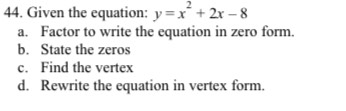### ¿Todavía tienes preguntas de matemáticas?

Pregunte a nuestros tutores expertos
Algebra
Pregunta44. Given the equation: $$y = x ^ { 2 } + 2 x - 8$$

a. Factor to write the equation in zero form.

b. State the zeros

c. Find the vertex

d. Rewrite the equation in vertex form.

$$y= (x- 2)(x+ 4)$$
$$x= 2, - 4\\(- 1, - 9)$$
$$y= (x+ 1)^2- 9$$